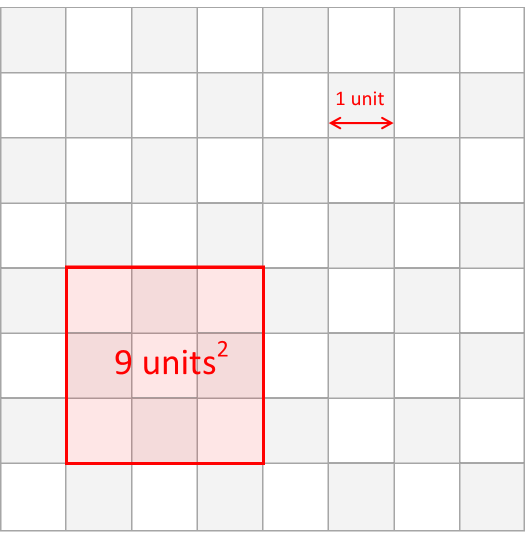# Ten square units

Last week, we looked at the puzzle of counting the number of squares on a chessboard.
This week, a quick little puzzle about how to make a square with an area of a non-perfect square number.If we have a regular chessboard, with each small square having an edge of one unit, it's pretty easy to see how we can make a square of 9 units2 (by selecting any region which is 3×3 in shape). The question is, how can we use the same chessboard to define a region of exactly 10 units2?
How can we make a square of 10 square units?